# Optics: Concave and Convex Mirrors and Lenses

This topic is part of the HSC Physics course under the section Ray Model of Light.

### HSC Physics Syllabus

• conduct a practical investigation to analyse the formation of images in mirrors and lenses via reflection and refraction using the ray model of light (ACSPH075)

In the fascinating world of physics, optics is the branch that studies the behaviour of light. Through this article, we'll explore how light interacts with mirrors and lenses, leading to image formation using the ray model of light.

### Mirrors vs Lenses

Mirrors and lenses are both fundamental tools in optics that manipulate light to produce images, but they do so through different mechanisms and have distinct properties. Here's a comparison of the two:

Mirrors

Mirrors reflect light. The image formed by a mirror is due to the reflection of light rays.

There are several types of mirrors

• Plane Mirrors: Produce a virtual image that is the same size as the object and is located as far behind the mirror as the object is in front of it.
• Concave Mirrors (Converging Mirrors): These are curved inwards. They can produce both real and virtual images, depending on the object's location.
• Convex Mirrors (Diverging Mirrors): These are curved outwards. They always produce virtual, diminished, and upright images.

Lenses

Lenses refract (or bend) light. The image formed by a lens is due to the refraction of light rays as they pass through the lens.

There are two types of lenses:

• Convex Lenses (Converging Lenses): Thicker in the middle than at the edges. They can produce both real and virtual images, depending on the object's location.
• Concave Lenses (Diverging Lenses): Thinner in the middle than at the edges. They always produce virtual, diminished, and upright images.

### The Ray Model of Light

The ray model simplifies light by treating it as a straight line, called a 'ray', moving in a specific direction. This is especially useful for geometric optics, where we're interested in how light interacts with surfaces rather than its wave-like properties.### What is a Principal Axis?

The principal axis of a lens or mirror is a straight line passing through the centre of the lens or mirror. For a spherical lens or mirror, this line is perpendicular to the surface of the lens or mirror at its centre. It provides a reference line for constructing ray diagrams and understanding how light interacts with the lens or mirror.

### What is a Focus?

The focus or focal point of a lens or mirror is a specific point where light rays either converge (come together) or appear to diverge (spread out) after interacting with the lens or mirror.

The distance between the lens or mirror and its focal point is termed the focal length.

The focal point has significant importance in optics as it's used for constructing ray diagrams and determining the nature, position, and size of the image formed by a lens or mirror.

### Concave MirrorsHow to draw ray diagrams for concave mirrors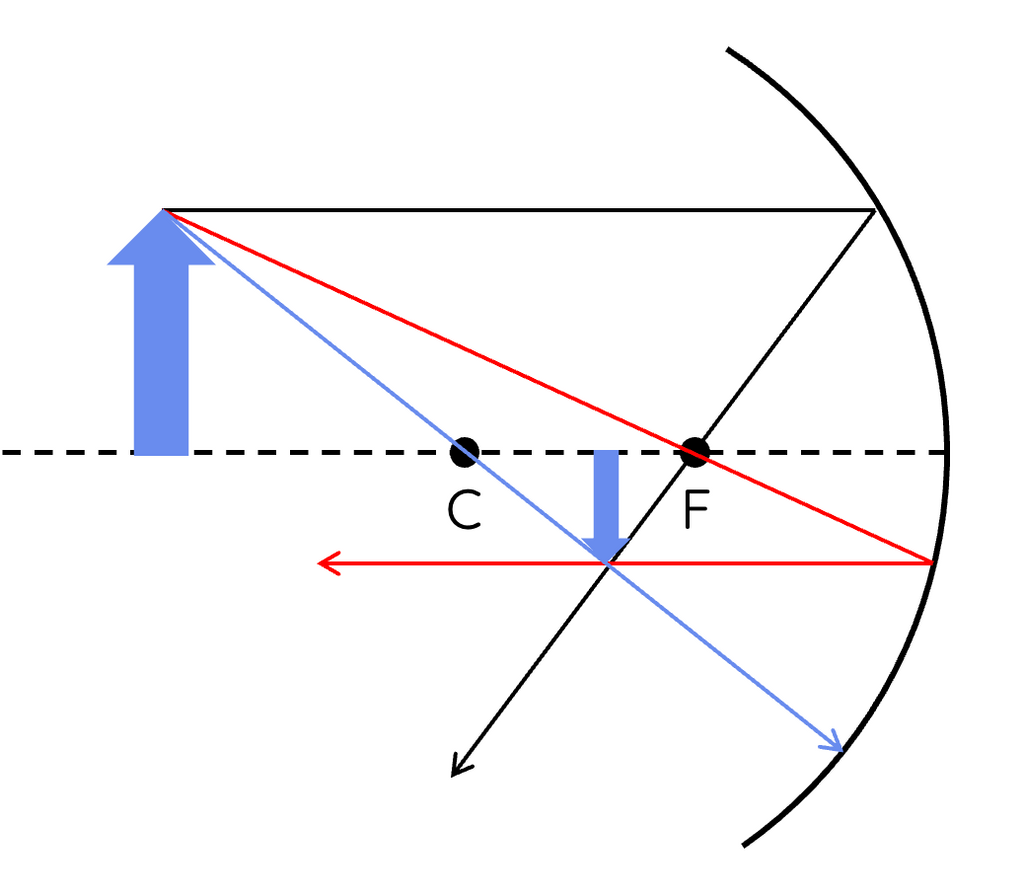1. Parallel Ray: Draw a ray parallel to the principal axis from the top of the object towards the mirror. After reflecting, this ray will pass through the focal point.

2. Focal Ray: Draw another ray from the top of the object, aiming directly at the focal point. After reflecting off the mirror, this ray will become parallel to the principal axis. The focus is the midpoint between the centre of curvature and the concave mirror.

3. Central Ray (optional for added accuracy): Draw a ray from the top of the object directly towards the centre of curvature. This ray will reflect back on itself.

Locate the Image:

After the rays have been drawn: where the reflected rays converge (or seem to converge) is where the top of the image will be. Draw a vertical arrow from this point down to the principal axis to represent the image.

Determine the nature of the image (real/virtual, upright/inverted, magnified/diminished) based on its position relative to the object and the principal axis.

• If the rays converge behind the concave mirror, the image formed will be a virtual image.
• If the rays converge in front of the concave mirror, the image formed will be a real image.

### What Type of Image Does a Concave Mirror Produce?

Concave mirrors can produce either real and inverted or virtual and upright images, depending on the object's position relative to the focal point.

• When the object is placed beyond the centre, an inverted, diminished and real image will be formed.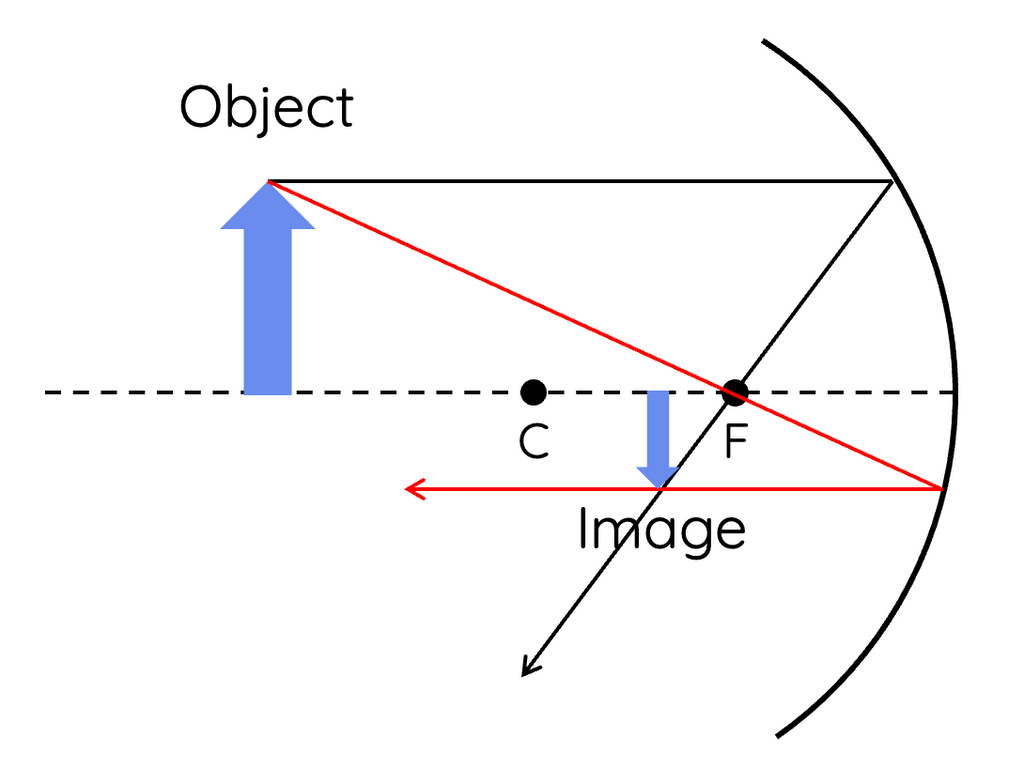• When the object is placed between the centre and the focus of the concave mirror, an inverted, magnified and real image will be formed.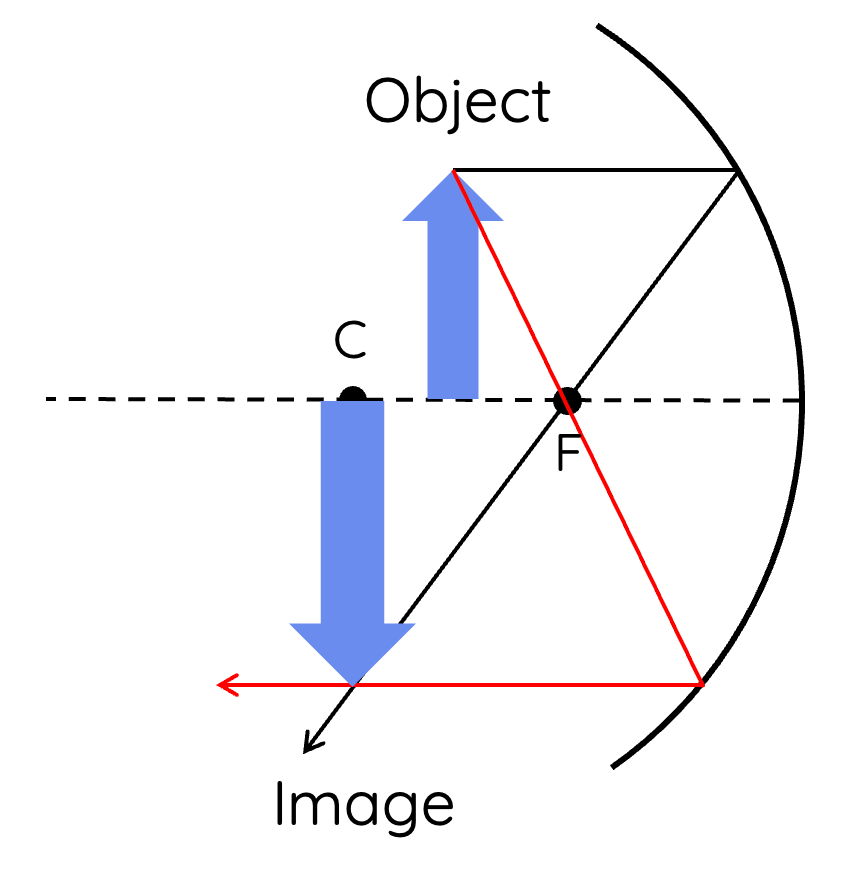• When the object is placed between the focus and the concave mirror, an upright, magnified and virtual image will be formed. In this case, a focal light ray cannot be drawn, so a central light ray is required to construct the image. The image is considered a virtual image because the reflected rays can only converge behind the mirror (shown by the dashed lines).### Convex Mirrors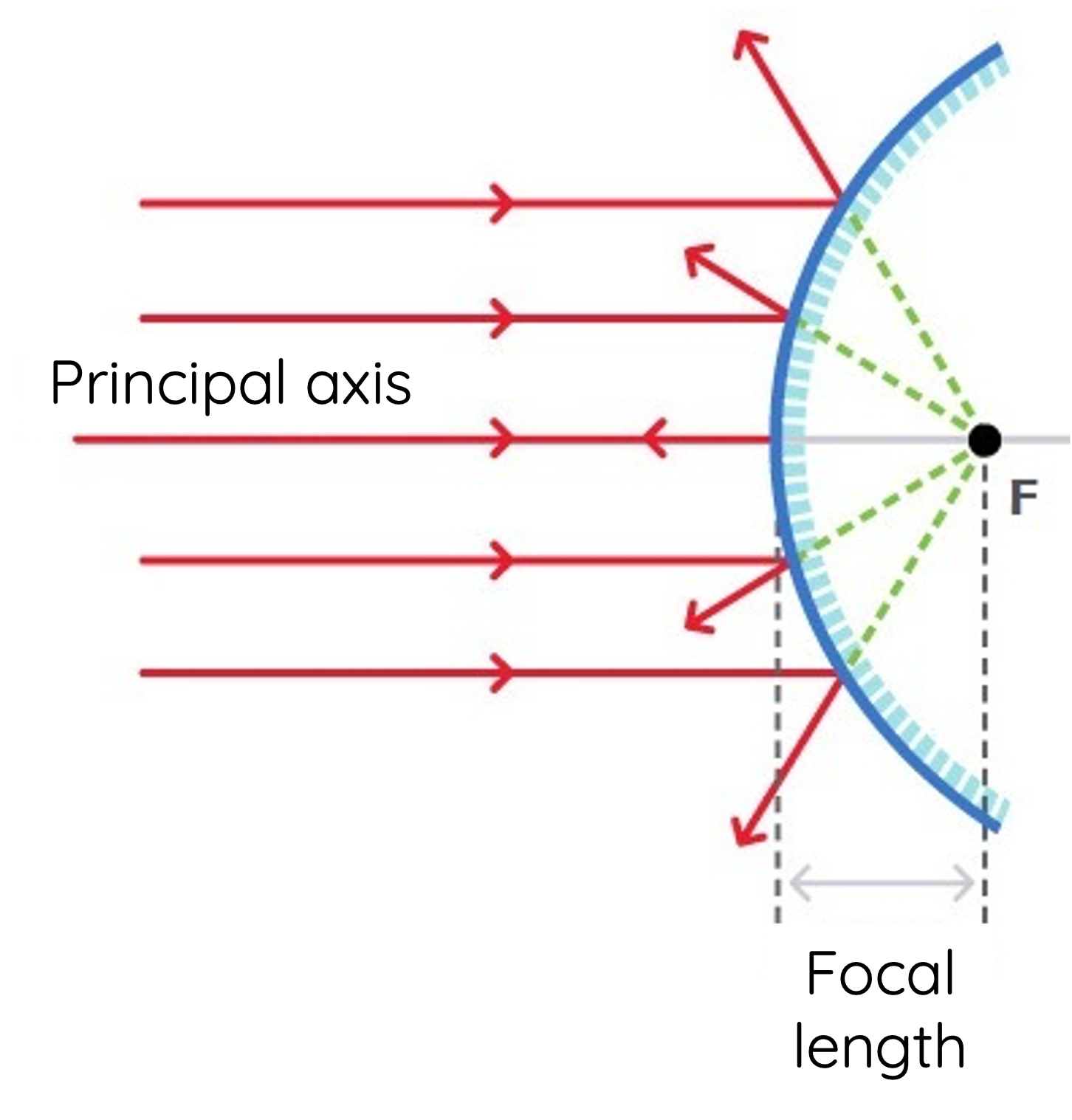• Image Characteristics: Convex mirrors always produce virtual, upright, and diminished.
• Ray Diagram: A common method is drawing a ray parallel to the principal axis (which reflects out as if it came from the focal point) and another ray aiming towards the focal point (reflecting parallel to the principal axis).

### Concave Lenses (diverging lenses)

A concave lens is thinner in the middle than at the edges. It causes parallel rays to diverge.

Steps for Drawing Ray Diagrams for Concave Lenses:

1. Parallel Ray: Start by drawing a ray parallel to the principal axis. After passing through the lens, this ray will diverge as if it originated from the focal point on the same side as the object.
2. Focal Ray: Draw another ray that heads toward the focal point on the opposite side of the lens. Upon hitting the lens, this ray will refract and exit the lens parallel to the principal axis.
3. Central Ray: Draw a ray that passes directly through the centre of the lens. This ray won't deviate and will continue straight through.
4. Image Formation: The rays will appear to diverge from a single point on the principal axis. That point gives the top of the virtual, upright image. Extend the base of the object to the principal axis to determine the image's complete position.

Image Characteristics: Always virtual, upright, and diminished.

• If the object is between the focus and the concave lens, the image will be virtual, upright (erect) and diminished.• If the object is between the focus and two focal lengths from the concave lens, the image will be virtual, upright and diminished.• If the object is beyond two focal lengths from the concave lengths, the image will be virtual, upright and diminished.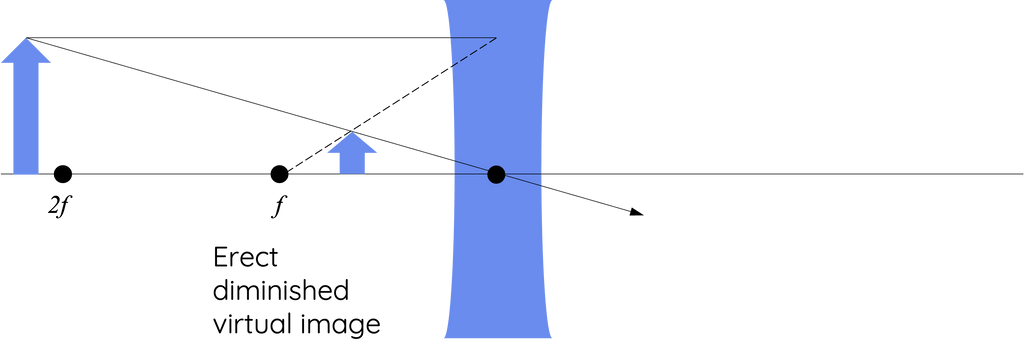### Convex Lenses (converging lenses)

A convex lens is thicker in the middle than at the edges. It can converge rays of light that are parallel to its principal axis.

Image Characteristics: Unlike concave lens, images formed via a convex lens can be real and inverted, or virtual and upright, depending on the object's position relative to the focal point. The position of the object also affects whether the image is magnified or diminished.

Steps for Drawing Ray Diagrams for Convex Lenses:

1. Parallel Ray: Begin by drawing a ray parallel to the principal axis. After passing through the lens, this ray will refract and pass through the focal point on the opposite side of the lens.

2. Focal Ray: Draw another ray that passes through the focal point on the side of the lens near the object. Upon entering the lens, this ray will refract and travel parallel to the principal axis.

3. Central Ray: Draw a ray that passes through the centre of the lens. This ray will continue in its original direction without deviating.

4. Image Formation: The point where all the refracted rays converge will give the top of the image. Extend the base of the object to the principal axis to complete the image.

• If the object is between the focal point and the convex lens, the image will be virtual, upright and magnified.• If the object is beyond the focal point and within two focal lengths from the lens, the image will be real, inverted and magnified.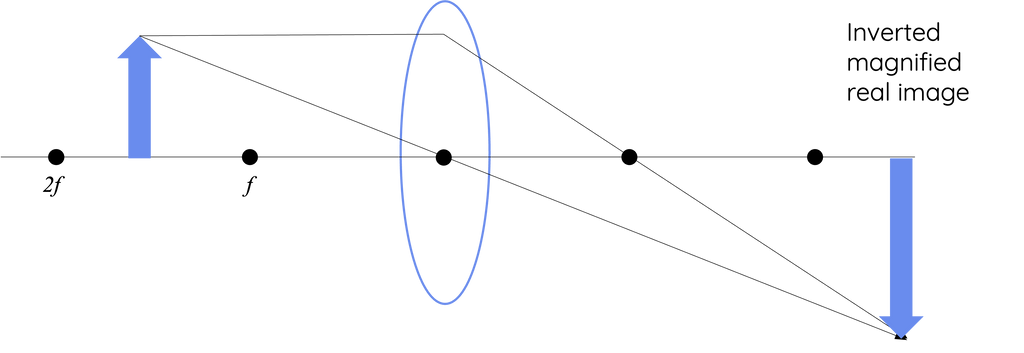• If the object is beyond two focal lengths from the lens, the image will be real, inverted and diminished.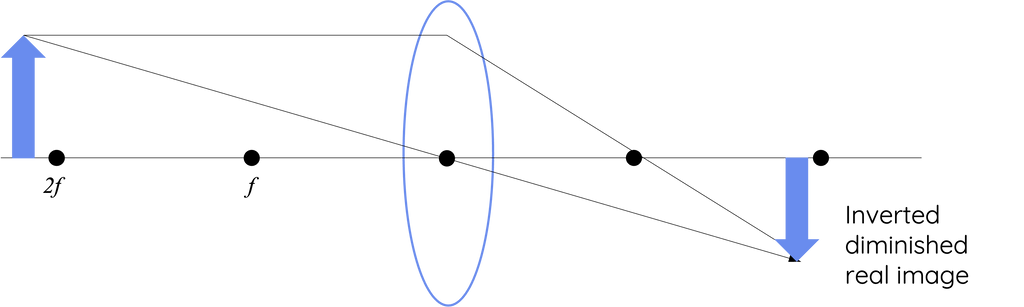### Mirror and Lens Equation

In optics, whether an image is virtual or real is often determined by the sign convention being used and the resulting signs of certain quantities in the lens or mirror equation.

The general lens/mirror equation is:

$$\frac{1}{f} = \frac{1}{v} + \frac{1}{u}$$

Where:

• f is the focal length of the lens or mirror.
• v is the image distance (distance from the lens or mirror to the image).
• u is the object distance (distance from the lens or mirror to the object).

This equation is not explicitly described in the HSC Physics syllabus. However, students are advised that knowing this equation may be required at the discretion of your school.

The sign conventions typically adopted are:

1. For mirrors:
• Distances measured in the direction of incident rays (towards the mirror) are taken as negative.
• Distances measured in the direction of reflected rays (away from the mirror) are taken as positive.
2. For lenses:
• Distances measured in the direction of incident rays (towards the lens) are taken as negative.
• Distances measured in the direction of refracted rays (away from the lens, or through the lens) are taken as positive.

The real versus virtual determination does not just depend on the sign of v. It's also essential to understand the type of optical device (concave vs. convex mirror, converging vs. diverging lens) and apply the appropriate sign conventions.